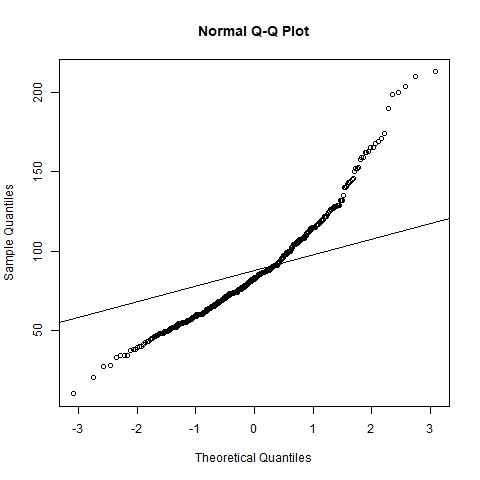# Descriptive statistics ¶

Go back

You need to come up with a model for your distribution. Use plots, functions, etc., and try to find as many properties as you can. This is the easiest part of the statistics (at least for non-mathematicians).

You will use normal plots, histograms, and box plots a lot. The first thing to do when having data in your hand would be to check the variables you are interested in with a histogram or a box plot. If you notice outliers with the boxplot (see next part), deal with it. You may notice something, and by cross-checking other diagrams, you may come up with ideas.

• look at the moments of your distribution
• check if your distribution is following a normal distribution (useful in tests)
• try to generate another distribution and compare it with your sample
• check if your variables are independent/dependant
• ...

## Outliers ¶

An outlier (valeurs anormales/aberrantes/extrêmes) is a value that looks out of place when observing your distribution. They are impacting your work a lot because they are impacting the mean.

Let's say you got some grades like 10,10,12 and your teacher made a mistake and submitted 10,10,120.

• mean(c(10,10,120)) = 46.66/20 (impacted a lot)
• median(c(10,10,120)) = 10/20 (not really impacted)

You can notice outliers easily with a boxplot, and you should remove/ignore/fix them. The median is not affected by outliers, so it's more robust.

## QQ-plot ¶

The Quantile-Quantile plot (QQ-plot) is a plot in which we are comparing the quantiles of our distribution with the quantile of another one. It is used to check if it's likely that our data is following a distribution.

qqnorm(ech$var) # qqnorm(ech$var, datax = TRUE)

# test with poisson distribution
qqline(rpois(100, mean(ech$var)))  We can see on this graph that it does not seem, that our sample is following a Poisson distribution.## Side note ¶ This is a hint, try to remember distribution properties because that might help. • we know that for a Poisson distribution, mean=variance=parameter • if we are observing a$mean=3$,$var \simeq 3$, then we could check a Poisson distribution with$\lambda=3\$
• this is not an accurate hint, just a hint as to how you could try to think (if you are lost, that is).